Guru

# If a, b, c and d are in proportion, prove that: (i) (5a + 7v) (2c – 3d) = (5c + 7d) (2a – 3b) (ii) (ma + nb): b = (mc + nd): d (iii)(a4 + c4): (b4 + d4) = a2c2: b2d2 (iv)(a2+ab) /(c2+cd) =(b2-2ab)/(d2-2cd) (v) (a+c)3 /(b+d) 3=a(a-c) 2/(b(b-d)2 (vi) a2+ab+b2/a2-ab+b2=c2+cd+d2/c2-cd+d2 (vii) (a2+b2) /(c2+d2) =ab+ad-bc/bc+cd-ad) (viii) abcd(1/a2+1/b2+1/c2+1/d2)=a2+b2+b2+c2)

• 0

This is a basic question from ML aggarwal book of class 10th, chapter 7, ratio and proportion., ICSE board

If a, b, c and d are in proportion,

We have to prove that:

(i) (5a + 7v) (2c – 3d) = (5c + 7d) (2a – 3b)

(ii) (ma + nb): b = (mc + nd): d

(iii)(a4 + c4): (b4 + d4) = a2c2: b2d2

(iv)(a2+ab) /(c2+cd) =(b2-2ab)/(d2-2cd)

(v) (a+c)3 /(b+d) 3=a(a-c) 2/(b(b-d)2 (vi) a2+ab+b2/a2-ab+b2=c2+cd+d2/c2-cd+d2

Question 19, 7.2

Share

1. Solution:

It is given that

a, b, c, d are in proportion

Consider a/b = c/d = k

a = b, c = dk

(i) LHS = (5a + 7b) (2c – 3d)

Substituting the values

= (5bk + 7b) (2dk – 3d)

Taking out the common terms

= k (5b + 7b) k (2d – 3d)

So we get

= k2 (12b) (-d)

= – 12 bd k2

RHS = (5c + 7d) (2a – 3b)

Substituting the values

= (5dk + 7d) (2kb – 3b)

Taking out the common terms

= k (5d + 7d) k (2b – 3b)

So we get

= k2 (12d) (-b)

= – 12 bd k2

Therefore, LHS = RHS.

(ii) (ma + nb): b = (mc + nd): d

We can write it as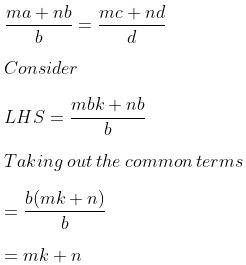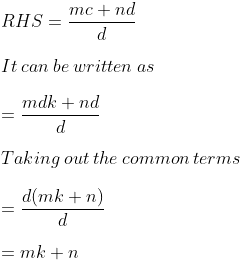Therefore, LHS = RHS.

(iii)(a4 + c4): (b4 + d4) = a2c2: b2d2

We can write it as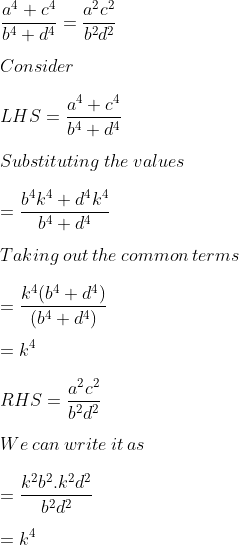Therefore, LHS = RHS.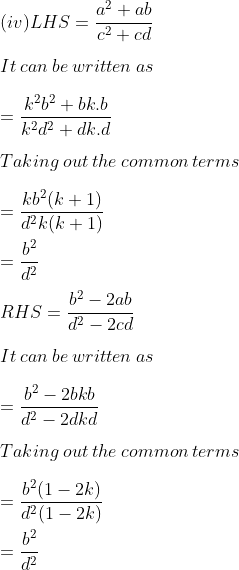Therefore, LHS = RHS.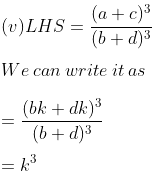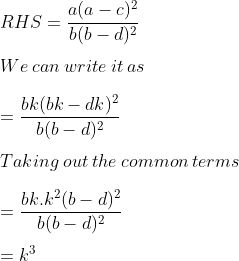Therefore, LHS = RHS.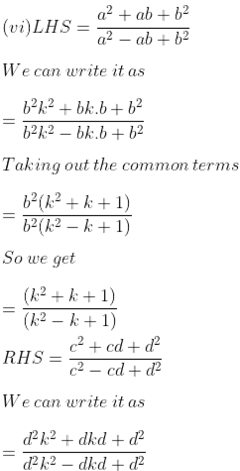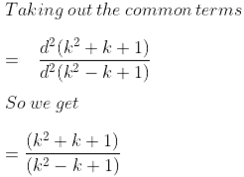Therefore, LHS = RHS.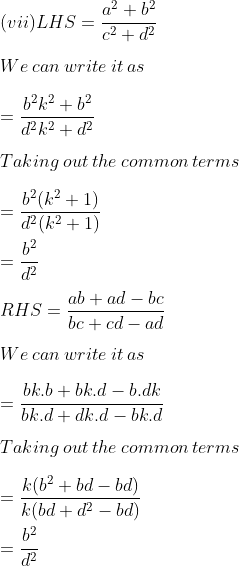Therefore, LHS = RHS.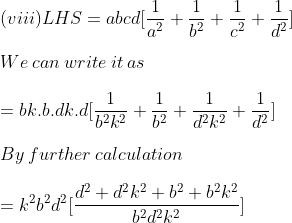So we get

= d2 (1 + k2) + b2 (1 + k2)

= (1 + k2) (b2 + d2)

RHS = a2 + b2 + c2 + d2

We can write it as

= b2k2 + b2 + d2k2 + d2

Taking out the common terms

= b2 (k2 + 1) + d2 (k2 + 1)

= (k2 + 1) (b2 + d2)

Therefore, LHS = RHS.

• 0